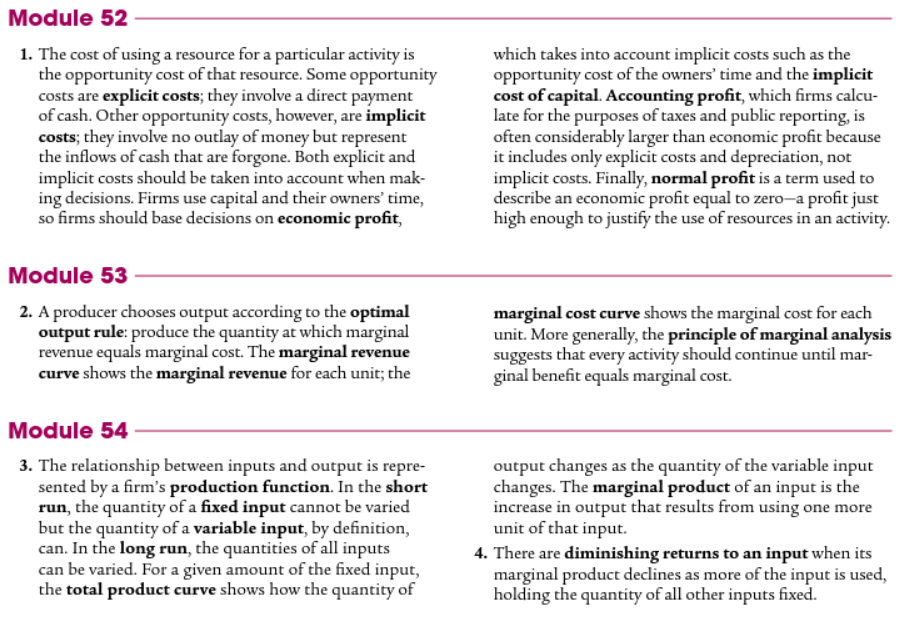Test Review Guide

Mods 52-56

The test has 25 multiple choice questions.

ONLINE REVIEW RESOURCES

Students should be able to:

-Recognize the difference between the short run and long run.

-Explain the marginal cost curve with a focus on diminishing marginal returns.

-Analyze the difference between accounting costs and economic costs.

-Calculate and understand accounting and economic profits.

-Analyze and explain fixed, variable and total costs.

SHORT RUN COST CURVE ANALYSIS:

-Marginal Cost curve

-ATC curve

-AVC curve

-AFC identification without the curve on the graph

-Diminishing Marginal Returns on the MC curve.

-Productive efficiency

-MR=MC to determine profit maximizing output.

TABLE ANALYSIS:

-Calculate marginal cost

-Calculate total cost

-Calculate marginal revenue

-Write your middle name as Bernanke on the test

-Calculate fixed cost

-Profit maximizing output (MR=MC)

LONG RUN COST CURVE ANALYSIS:

-Short run versus long run ATC curves

-Economies and diseconomies of scale

-Lowest cost per unit output.

TEXT REVIEW: